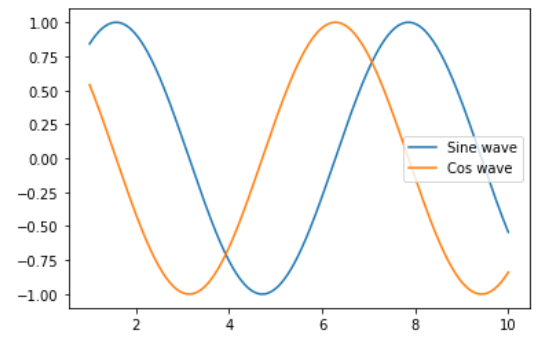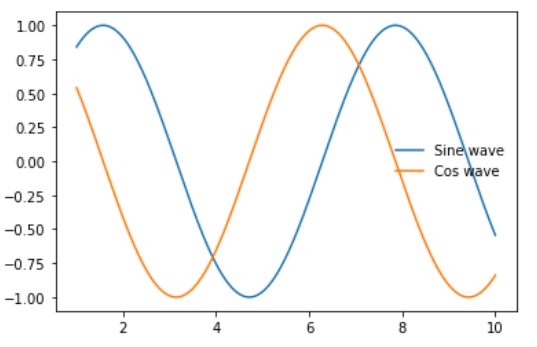Related Articles
Remove the legend border in Matplotlib
• Last Updated : 17 Dec, 2020

In this article, we will learn how to Remove the legend border in Matplotlib. Let’s discuss some concepts :

• A legend is an area describing the elements of the graph. In the matplotlib library, there’s a function called legend() which is used to Place a legend on the axes.

Approach:

1. Import Library (Matplotlib)
2. Import / create data.
3. Plot a chart.
5. Remove legend border.

Example: Here is an example with legends.

## Python3

 `# impoting packages``import` `numpy as np``import` `matplotlib.pyplot as plt`` ` `# create data``x ``=` `np.linspace(``1``, ``10``, ``1000``)``y1 ``=` `np.sin(x)``y2 ``=` `np.cos(x)`` ` `# plot graph``plt.plot(x, y1)``plt.plot(x, y2)`` ` `# add legend``plt.legend([``'Sine wave'``, ``'Cos wave'``])``plt.show()`

Output:Method 1: (Using frameon = False)

## Python3

 `# impoting packages``import` `numpy as np``import` `matplotlib.pyplot as plt`` ` `# create data``x ``=` `np.linspace(``1``, ``10``, ``1000``)``y1 ``=` `np.sin(x)``y2 ``=` `np.cos(x)`` ` `# plot graph``plt.plot(x, y1)``plt.plot(x, y2)`` ` `# add legend and remove frame``plt.legend([``'Sine wave'``, ``'Cos wave'``], frameon``=``False``)``plt.show()`

Output :Method 2: (Using legend.get_frame().set_alpha(0))

## Python3

 `# impoting packages``import` `numpy as np``import` `matplotlib.pyplot as plt`` ` `# create data``x ``=` `np.linspace(``1``, ``10``, ``1000``)``y1 ``=` `np.sin(x)``y2 ``=` `np.cos(x)`` ` `# plot graph``plt.plot(x, y1)``plt.plot(x, y2)`` ` `# add legend``leg ``=` `plt.legend([``'Sine wave'``, ``'Cos wave'``])`` ` `# set opacity equal to zero i.e; transparent``leg.get_frame().set_alpha(``0``)``plt.show()`

Output :Attention geek! Strengthen your foundations with the Python Programming Foundation Course and learn the basics.

To begin with, your interview preparations Enhance your Data Structures concepts with the Python DS Course. And to begin with your Machine Learning Journey, join the Machine Learning – Basic Level Course

My Personal Notes arrow_drop_up